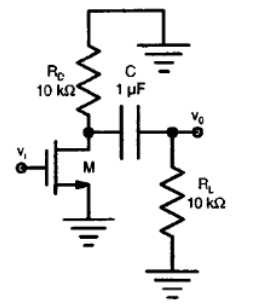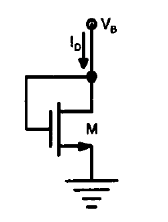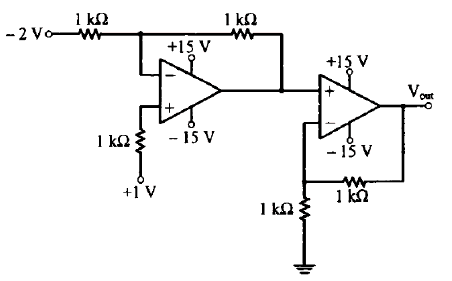## Monday, 11 March 2013

### Video Solutions for GATE 2013 EC : Analog Circuits - Two Mark Questions

1. The ac schematic of an NMOS common source stage is shown below, where part of the biasing circuit has been omitted for simplicity. For an N-channel MOSFET ‘M’, the transconductance gm = 1 mA/Volt, and body effect and Channel length modulation effect are to be neglected. Then the lower cutoff frequency in Hz of the circuit is approximately at _______.A.  8          B.  32              C.  50                          D.  200

Ans :  A1. The small signal resistance ( i.e. dVB/dID ) in KΩ offered by an N- channel MOSFET ‘M’ shown in fig., at a bias point of VB = 2 volts is _______.( Assume Knn.Cox(W/L) = 40 μA/V2 and VT = 1 volt, and neglect body effect and channel length modulation effect. )A.  12.5                 B.  25               C.  50                          D.  100

Ans :  B1. In the circuit shown below, assume ideal op-amps. Then Vout in volts is _____A.  4                      B.  6                 C.  8                D.  10

Ans :  C